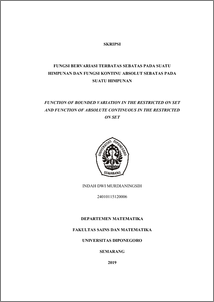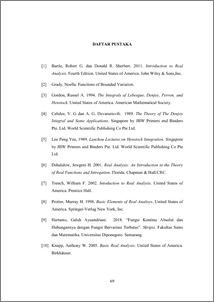# FUNCTION OF BOUNDED VARIATION IN THE RESTRICTED ON SET AND FUNCTION OF ABSOLUTE CONTINUOUS IN THE RESTRICTED ON SET

Murdianingsih, Indah Dwi (2019) FUNCTION OF BOUNDED VARIATION IN THE RESTRICTED ON SET AND FUNCTION OF ABSOLUTE CONTINUOUS IN THE RESTRICTED ON SET. Undergraduate thesis, UNDIP.Preview
PDF
650KbPDF
Restricted to Repository staff only

841KbPreview
PDF
84Kb

## Abstract

Function of bounded variation in the restricted on set are denoted by BV* . BV* functions is a function with finite strong variation. Every BV* function is bounded variation functions, and in the interval BV* functions equals bounded variation functions. Some properties of function are applied in BV* functions; there is multiplication with scalar, sum of two functions, and product of two functions. Every BV* functions on E is also a BV* functions on E . Function of absolute continuous in the restricted on set are denoted by AC* . AC* functions is a function that is stronger than absolutely continuous functions. Every AC* function is absolutely continuous function, and in the interval AC* functions equals absolutely continuous functions. Some properties of AC* function are multiplication with scalar, absolute value of function, sum of two functions, and product of two functions. Every AC* function on E is a AC* functions on any subset of E . Every AC* function is BV* functions. This final discusses about BV* function and AC* function. Keywords: function of bounded variation in the restricted on set, function of absolute continuous in the restricted on set.

Item Type: Thesis (Undergraduate) Q Science > QA Mathematics Faculty of Science and Mathematics > Department of Mathematics 84264 INVALID USER 13 Jun 2022 10:39 13 Jun 2022 10:39

Repository Staff Only: item control page